• 高斯滤波器matlab实现 function h = d2gauss(n1,std1,n2,std2,theta)，还有主函数和一些具体实例
• 图像颜色空间转换及matlab实现、图像噪声概率密度分布与MATLAB实现、理想滤波器设计与MATLAB实现、巴特沃斯滤波器设计与MATLAB实现高斯滤波器设计与MATLAB实现
• 本资源是基于matlab高斯滤波器仿真，分析了高斯脉冲成形滤波器对矩形脉冲输入信号的影响。矩形脉冲通过高斯脉冲成形滤波器后变成了高斯脉冲，有效的改变矩形突变的上升沿和下降沿。
• 论文的matlab实现Varghese、贾斯汀等。 “用于从数字图像中去除周期性噪声的自适应高斯陷波滤波器” IET 图像处理，工程技术学院 (IET)，2020 年 3 月https://doi.org/10.1049/iet-ipr.2018.5707 请在您使用它的...
• sigama 自适应高斯滤波器, matlab 程序，里边有相应的注释和测试数据，自己可以试试
• matlab实现的2D高斯带通滤波器，支持2D时间序列滤波。
• ## matlab高斯滤波器

万次阅读 热门讨论 2017-07-26 16:00:33
1、使用matlab自带的函数 %%%%%%%%%%%%%%%%%%%%%%%%%%%%%%%%%%%%%%%%%%%%%%%%%% clear; img = imread('lena.tif'); w = fspecial('gaussian',[5,5],1); %replicate:图像大小通过赋值外边界的值来扩展 %...
1、使用matlab自带的函数

%%%%%%%%%%%%%%%%%%%%%%%%%%%%%%%%%%%%%%%%%%%%%%%%%%
clear;

w = fspecial('gaussian',[5,5],1);
%replicate:图像大小通过赋值外边界的值来扩展
%symmetric 图像大小通过沿自身的边界进行镜像映射扩展
I11 = imfilter(img,w,'replicate');
figure(1);
imshow(img);title('原图像');
figure(2);
imshow(I11);title('matlab高斯滤波后的图像');
结果：可以看到帽子和头发都非常显著的平滑了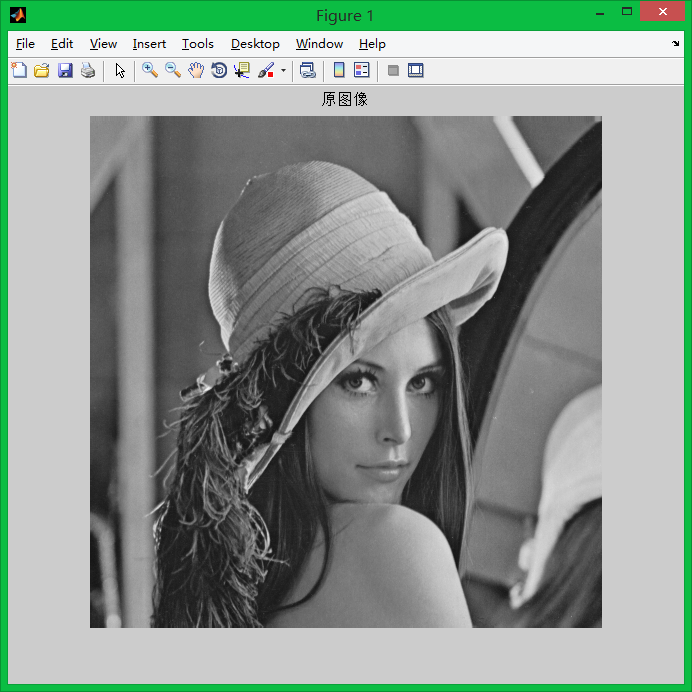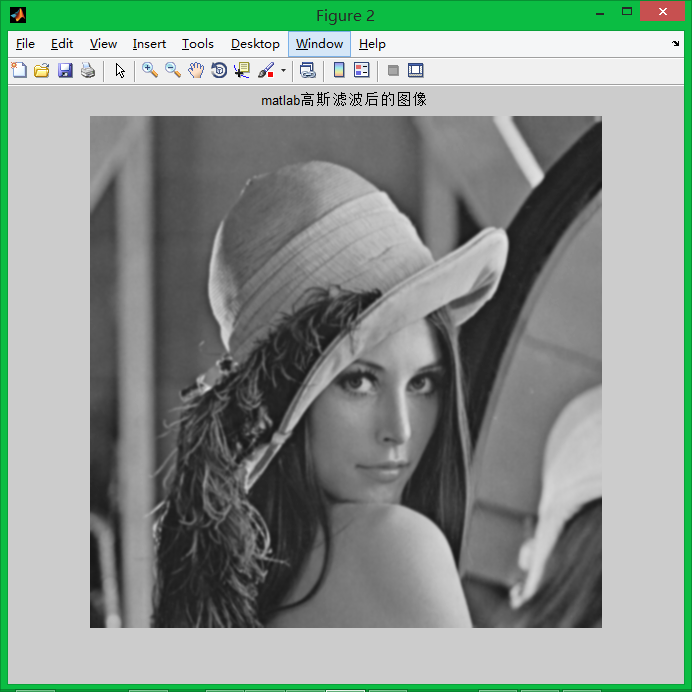2、自己编写的高斯滤波函数
%own gaussian function
I = double(I);%convert unit8 to double
myimg = I;
[rows, cols] = size(I);
size = 5;
conv = zeros(size,size);%高斯核
segma = 1;
sum = 0;
center  = 3;
for i=1:size
for j=1:size
%%利用高斯函数计算模板的各个参数
temp = exp ( -((i-center)*(i-center) + (j-center)*(j-center)) / 2*segma*segma );
conv(i,j) = temp / (2*3.14*segma*segma);
sum =sum +  conv(i,j);
end
end
conv = conv ./ sum;
disp('kernel');
display(conv);
%use gaussian kernel to smooth image
for k=1:rows
for m= 1:cols
sum =0;
%%卷积：模板与图像对应部分相乘再累加
for i=1:size
for j=1:size
if( ( (k-center+i) > 0 && (k-center+i) <= rows ) && ((m-center+j)>0 && (m-center+j)<=cols) )
sum = sum + conv(i,j) * I(k-center+i, m-center+j);
end
end
end
%%替换掉原像素
myimg(k,m) = sum;
end
end
figure(3);
imshow(myimg,[]);
title('Gaussion Image');结论：使用自己编写的滤波，虽然也起到了平滑效果，但是图像亮度偏高些，还不知道原因，希望大神指点指点。
展开全文• 二维高斯滤波器图像滤波MTATLAB程序设计 用MATLAB设计一个33模板标准差为的二维高斯低通滤波器(Gaussion low pass filter分别对灰度图像真彩色图像伪彩色图像进行滤波处理 %二维高斯低通滤波器 %文件为glpf.m ...
• 函数输入： - filter_size: 过滤器的大小- 西格玛：标准偏差函数输出： - 二维高斯滤波器矩阵在 3D 中绘制滤波器矩阵的示例： g1=Gaussian_filter(50,2); g2=Gaussian_filter(50,7); g3=Gaussian_filter(50,11); 图1...
• 频域滤波器器的一些相关实验和滤波器实现（包括理想低通，Butterworth低通，高斯低通，理想高通，Butterworth高通，高斯高通滤波器
• 版权声明：<–本博客所有内容均为自己在学习工作中的总结、摘录等-- --转载请注明出处-- --如有侵权请联系删除–>...%GULS 高斯低通滤波器,D0为截至频率的（相当于设置在傅里叶谱图的半径值）...
版权声明：<–本博客所有内容均为自己在学习工作中的总结、摘录等-- --转载请注明出处-- --如有侵权请联系删除–> https://mp.csdn.net/mdeditor/89389365#
function [g] =GaussianHighPassFilter (image_input , D0)
%GULS 高斯高通滤波器,D0为截至频率的（相当于设置在傅里叶谱图的半径值）
%判断图像是否为灰度图，不是灰度图的话，将图像变为灰度图
f = image_input;
f = mat2gray(f,[0 255]);
% 1.给定一幅大小为M*N的输入图像f(x,y),得到填充参数P = 2M,Q = 2N
[m,n] = size(f);
P = 2 * m;
Q = 2 * n;
% 2.对f(x,y)添加必要数量的0，形成大小为P * Q的填充后的图像fp(x,y)
fp = zeros(P,Q);
fp(1:m,1:n) = f(1:m,1:n);
% 3.用(-1)^(x+y)乘以fp(x,y)移到其交换的中心
for i = 1 : m
for j = 1 : n
fp(i,j) = double(fp(i,j)*(-1)^(i+j));
end
end
% 4.计算来自步骤3的图像的DFT，得到F(u,v)
F = fft2(fp,P,Q);
% 5.生成一个实的、对称的滤波函数H(u,v),其大小为P*Q，中心在（P/2,Q/2）处。
% 用阵列相乘形成乘积G(u,v) = H(u,v)F(u,v);即G(i,k)=H(i,k)F(i,k)
H = zeros(P,Q);
a = 2 * D0^2;
for u = 1 :P
for v = 1:Q
D = (u-(m+1.0))^2+(v-(n+1.0))^2;
H(u,v) = 1 - exp((-D)/a);
end
end
G = H.*F;
% 6.得到处理后的图像
gp = ifft2(G);
gp = real(gp);
for i = 1 : m
for j = 1 : n
gp(i,j) = double(gp(i,j)*(-1)^(i+j));
end
end
% 7.通过从gp(x,y)的左上象限提取M*N区域，得到最终处理结果g(x,y)
g(1:m,1:n) = gp(1:m,1:n);


结果图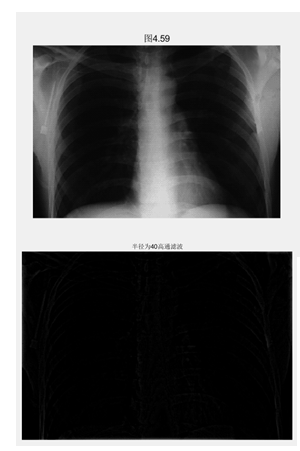展开全文• 图像处理中用于噪声过滤的高斯滤波器。 图像处理中使用的滤波器的二阶导数 高斯的 LOG 拉普拉斯算子 这是 gaussina 的二阶导数，用于边缘检测和平滑图像，从而减少噪声的影响。 使用符号数学工具箱计算的导数。 现在...
• 使用高斯高通滤波器的同态滤波的简单matlab实现
• 修改后的非局部均值和双边滤波器Matlab代码实现，如I. Frosio，J。Kautz，统计最近的图像去噪邻居，IEEE Trans中所述。 图像处理，2018年。该存储库还包含Matlab代码，用于复制本文中描述的玩具问题的结果。 执照 ...
• 高斯滤波器是空间滤波器的一种，学习高斯滤波器，需要先了解一下空间滤波的知识。 高斯滤波器是一种平滑线性滤波器，使用高斯滤波器对图像进行滤波，其效果是降低图像灰度的“尖锐”变化，也就是使图像“模糊”了...
        高斯滤波器是空间滤波器的一种，学习高斯滤波器，需要空间滤波的知识作为先验知识（各种数字图像处理的书中都有介绍，冈萨雷斯的经典教材说的还是比较清楚的，建议看英文版，个人感觉比翻译过来的更容易理解）。

高斯滤波器是一种平滑线性滤波器，使用高斯滤波器对图像进行滤波，其效果是降低图像灰度的“尖锐”变化，也就是使图像“模糊”了。高斯滤波对于抑制服从正态分布的噪声效果非常好，其代价是使图像变得“模糊”。当然，有时对图像进行平滑滤波的目的就是让图像变得模糊。

高斯滤波的模板是用高斯公式计算出来的。下面是一维和二维高斯分布函数及其函数图像：

一维高斯分布函数：                                     二维高斯分布函数：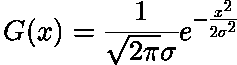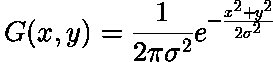它们的图像分别为：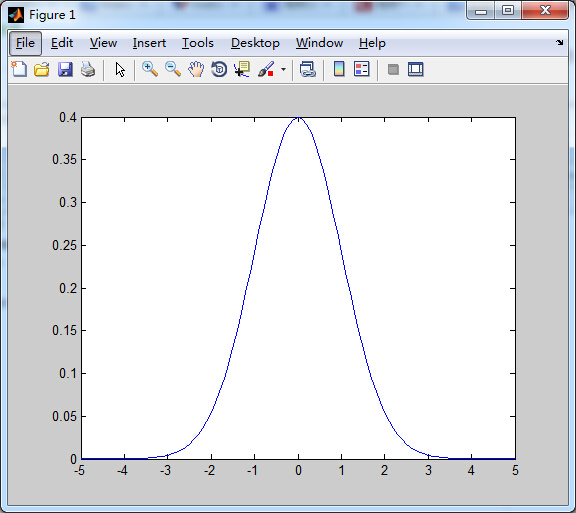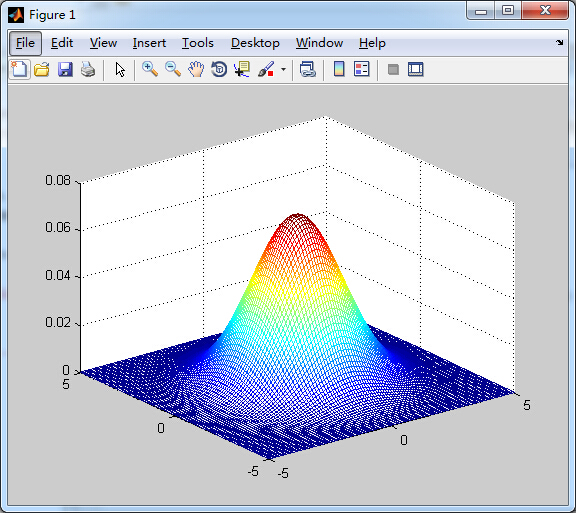那么高斯滤波模板是怎么生成的呢？是通过二维高斯函数计算出来的。例如，我们假设一个高斯模板的长、宽均为5，方差为0.5，那么首先，我们要在模板上建立一个坐标系，其原点就是高斯模板的中心点，如下图：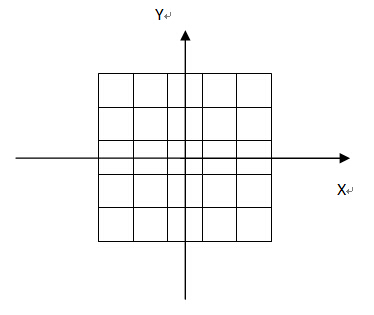然后每个模板的坐标就可以确定了：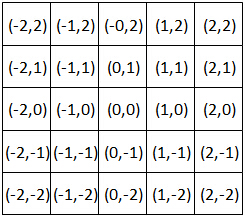每个格子对应的坐标，就是二维高斯分布中的（x，y）坐标的值。现在，我们可以计算出高斯模板上每个坐标位置的系数了。例如：
（-2,2）上的权值：W =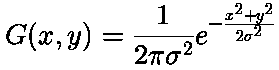，把pi = 3.14，  σ=0.5，x=-2，y=2带入上式，获得（-2,2）处的权值。
同理，可以计算 其他位置的权值。
最后获得一个5*5，标准差σ为0.5的高斯滤波器（经过归一化以后）：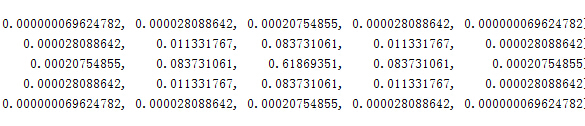matlab中可以使用fspecial函数实现高斯滤波模板，使用方法为：YourFilter = fspecial('gaussian', [ 5 5], 0.5);
在matlab中可以查看fspecial的源代码，通过open fspecial打开。我之前自己写了一个matlab版的实现高斯模板的代码，通过与fspecial的源代码对比，有些不一样的地方，而且我的代码确实不如自带的快，所以在这里只贴源代码。
fspecial函数可以实现多种类型的滤波器，当第一个参数为"gaussian'时，实现的是高斯滤波器，源代码通过switch判断用户想得到哪种类型的滤波器，这里只把与高斯滤波器有关的代码贴出来，并放在一个函数中，我们把这个函数命名为gaussianFilter。函数的代码如下：

function h = gaussianTemplate2( p2, p3 )
%p2是模板的大小，以[m n]的形式定义
%p3是标准差，即σ
%h是输出的高斯模板
%以下代码是从fspecial中贴出来的代码

siz   = (p2-1)/2;           %siz为模板行与列各自最大的坐标值
std   = p3;                 %标准差幅值给std

%通过meshgrid产生模板上所有位置的x和y的值，这样的好处是可以避免使用循环去
%变换坐标值，提高程序运行的速度
[x,y] = meshgrid(-siz(2):siz(2),-siz(1):siz(1));

%二维高斯分布公式中e的指数
arg   = -(x.*x + y.*y)/(2*std*std);

%这里没有乘以1/2*pi*σ^2，也就是没有乘以e前面的系数，因为后面在作归一化的
%时候，会把这个系数约去，所以在这里直接省去乘法运算，提高程序运行速度
h     = exp(arg);

%这句话没有太理解，貌似只有在max(h(:))大于1的时候h才有可能小于eps*max(h(:))
%个人理解应该是限制一下h中的权值，如果权值相对于h中的最大值特别小的话，可以
%忽略不计，使之为零
h(h<eps*max(h(:))) = 0;

%归一化
sumh = sum(h(:));
if sumh ~= 0,
h  = h/sumh;
end;
end        获得高斯模板后，使用imfilter函数对图像进行滤波，即可获得高斯滤波效果。下面我们使用不同的高斯滤波器，对同一幅图像进行滤波，滤波的结果如下所示：原始图像                                                           加入高斯噪声图像使用5*5，方差为0.5的高斯滤波器滤波                             使用5*5，方差为1.5的高斯滤波器滤波使用11*11，方差为0.5的高斯滤波器滤波
展开全文图像处理
• matlab开发-用于图像处理的高斯带通滤波器。此函数在频域中执行高斯带通滤波。
• ## matlab实现图像的高斯滤波

万次阅读 多人点赞 2019-04-23 16:09:08
高斯滤波的含义：高斯滤波就是对整幅图像进行加权平均的过程，每一...高斯滤波的作用：高斯滤波是一种线性平滑滤波，适用于消除高斯噪声，广泛应用于图像处理的减噪过程。 高斯滤波函数 一维高斯分布 二维高斯分...
转载地址：https://blog.csdn.net/lvquanye9483/article/details/81592574
高斯滤波的含义：高斯滤波就是对整幅图像进行加权平均的过程，每一个像素点的值，都由其本身和邻域内的其他像素值经过加权平均后得到。
高斯滤波的作用：高斯滤波是一种线性平滑滤波，适用于消除高斯噪声，广泛应用于图像处理的减噪过程。
高斯滤波函数
一维高斯分布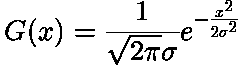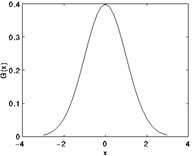二维高斯分布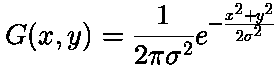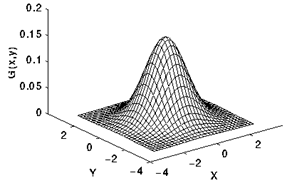高斯核
理论上，高斯分布在所有定义域上都有非负值，这就需要一个无限大的卷积核。实际上，仅需要取均值周围3倍标准差内的值，以外部份直接去掉即可。如下图为一个标准差为1.0的整数值高斯核。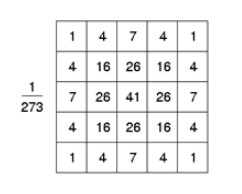高斯滤波的卷积运算
假设有一个卷积核h，就一般为3*3的矩阵：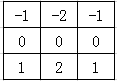有一个待处理矩阵x：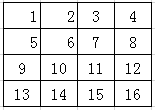h*x的计算过程分为三步
第一步，将卷积核翻转180°，也就是成为了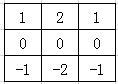第二步，将卷积核h的中心对准x的第一个元素，然后对应元素相乘后相加，没有元素的地方补0。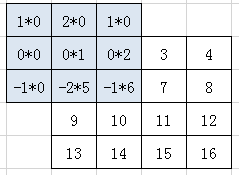这样结果Y中的第一个元素值Y11=1*0+2*0+1*0+0*0+0*1+0*2+-1*0+-2*5+-1*6=-16 第三步每个元素都像这样计算出来就可以得到一个输出矩阵，就是卷积结果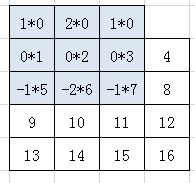最后结果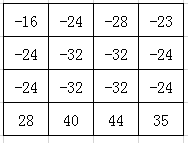高斯滤波的性质
高斯函数具有五个重要的性质，这些性质使得它在早期图像处理中特别有用．这些性质表明，高斯平滑滤波器无论在空间域还是在频率域都是十分有效的低通滤波器，且在实际图像处理中得到了工程人员的有效使用．高斯函数具有五个十分重要的性质，它们是： 　　（1）二维高斯函数具有旋转对称性，即滤波器在各个方向上的平滑程度是相同的．一般来说，一幅图像的边缘方向是事先不知道的，因此，在滤波前是无法确定一个方向上比另一方向上需要更多的平滑．旋转对称性意味着高斯平滑滤波器在后续边缘检测中不会偏向任一方向． 　　（2）高斯函数是单值函数．这表明，高斯滤波器用像素邻域的加权均值来代替该点的像素值，而每一邻域像素点权值是随该点与中心点的距离单调增减的．这一性质是很重要的，因为边缘是一种图像局部特征，如果平滑运算对离算子中心很远的像素点仍然有很大作用，则平滑运算会使图像失真． 　　（3）高斯函数的傅立叶变换频谱是单瓣的．正如下面所示，这一性质是高斯函数付立叶变换等于高斯函数本身这一事实的直接推论．图像常被不希望的高频信号所污染(噪声和细纹理)．而所希望的图像特征（如边缘），既含有低频分量，又含有高频分量．高斯函数付立叶变换的单瓣意味着平滑图像不会被不需要的高频信号所污染，同时保留了大部分所需信号． 　　（4）高斯滤波器宽度(决定着平滑程度)是由参数σ表征的，而且σ和平滑程度的关系是非常简单的．σ越大，高斯滤波器的频带就越宽，平滑程度就越好．通过调节平滑程度参数σ，可在图像特征过分模糊(过平滑)与平滑图像中由于噪声和细纹理所引起的过多的不希望突变量(欠平滑)之间取得折衷． 　　（5）由于高斯函数的可分离性，较大尺寸的高斯滤波器可以得以有效地实现．二维高斯函数卷积可以分两步来进行，首先将图像与一维高斯函数进行卷积，然后将卷积结果与方向垂直的相同一维高斯函数卷积．因此，二维高斯滤波的计算量随滤波模板宽度成线性增长而不是成平方增长．
matlab代码：
clear,clc;

% 读取图像
M = size(Img);
if numel(M)>2
gray = rgb2gray(Img);
else
gray = Img;
end

% 创建滤波器
W = fspecial('gaussian',[5,5],1);
G = imfilter(gray, W, 'replicate');
figure(1);
subplot(121); imshow(gray); title('原始图像');
subplot(122); imshow(G);    title('滤波后图像');


展开全文• 这是一个包括程序和文档在内的高斯低通滤波器的设计与实现过程，对于滤波器初学者很有用。
• %GULS 高斯低通滤波器,D0为截至频率的（相当于设置在傅里叶谱图的半径值） %判断图像是否为灰度图，不是灰度图的话，将图像变为灰度图 f = image_input; f = mat2gray(f,[0 255]); % 1.给定一幅大小为M*N的输入图像f...
• 致谢：此文件的灵感来自 Douglas Lanman 在http://www.mathworks.com/matlabcentral/fileexchange/9645-steerable-gaussian-filters 上的“可操纵高斯滤波器” 然而，Douglas Lanman 的实现有两个主要改进： 1....
• ISO 16610-21 开放轮廓高斯滤波器该库包含根据 ISO 16610-21:2011 实现开放轮廓高斯卷积滤波器的函数。 该滤波器旨在用于将短波和长波分量轮廓与表面计量学中的主表面轮廓分离。 粗糙度参数可以从短波分量轮廓进行...
• ISO 16610-21 封闭轮廓高斯滤波器该库包含根据 ISO 16610-21:2011 实现闭合轮廓高斯卷积滤波器的函数。 该滤波器旨在用于从表面计量学中的主要表面轮廓中分离短波和长波分量轮廓。 粗糙度参数可以从短波分量轮廓进行...
• matlab开发-用于图像处理的高斯带通滤波器。此函数在频域中执行高斯带通滤波。Matlab development - Gaussian band-pass filter for image processing. This function performs Gaussian band-pass filtering in the...
• 高通滤波器matlab实现，实现高斯 理想 巴特的高通滤波器实现
• 1 高斯滤波器简介 高斯滤波器是一种线性滤波器，能够有效的抑制噪声，平滑图像。其作用原理和均值滤波器类似，都是取滤波器窗口内的像素的均值作为输出。但其窗口模板的系数和均值滤波器不同，均值滤波器的模板系数...
• 用于对时间序列数据的高斯滤波。是m文件，需要在matlab中操作。...

# 高斯滤波器matlab实现matlab 订阅